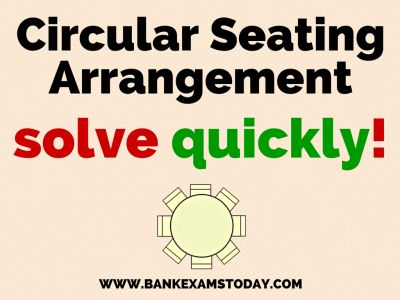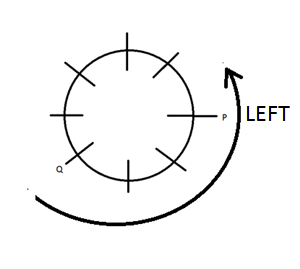# Circular Seating Arrangement Facing Outside and Inside

Seating arrangements is an important part of Reasoning section of Banking exams. Few years back simple questions were asked from this part but now in every exam there is Facing Outside and Inside Seating Arrangement sets. Today I am going to share basics technique to solve these questions.## Facing centre

It means that all the people sitting in the circular arrangements are sitting keeping their face towards the centre.Towards left: It means anti clockwise direction.

Let us understand it through an example. P sits third to the left of Q.In the circle you can sit P anywhere .Towards Right: Clock wise direction

For example P sits 3rd to the right of B## Facing towards OUTSIDE

Towards Left: In this case, the scenario will entirely change which means it would become clockwise direction

For example P sits third the left of Q.Towards Right:Here, again the direction would become anticlockwise

P sits third to the right of Q### Now let us do some question:

P, Q, R,S, T , U, V, W are sitting around a circular table facing centre. P sits third to the right of W and third to the left of Q.S sits second to the right of T, V sits second to the left of R . T is not the neighbor of Q while U is not the neighbor of T nor W.

Solution:

P sits third to the left of Q. T is not the neighbor of Q. S sits second to the right of THighlighted positions can not have T.U is not the neighbor of Q or WHighlighted places can not have U

V sits second to left of R.

### Q1.

Who sits second to the left of V.

Ans. U

### Q2.

Who sits between U and P.

Ans. S

### Q3.

Starting from P’s position if all the people are arranged in alphabetical order in clock wise direction sitting positions of how many members would not change excluding P?

Ans. 1

Starting from P and moving in clockwise direction we will have       a structure like:

P    Q      R       S      T      U    V

P     T      R     W     V     Q      S

We can see that position of R only does not change. Remember we need to exclude P.

### Q4.

Which of the  following pairs have only person sitting between them if counting is done clockwise direction?
1. T, V
2. V, Q
3. W,P
4. R,P
5. None

Explanation:
If you move in clockwise direction
Between  T and V =  two persons ( W and R)
Between V and Q = 0
Between W and P =4 persons (V, Q, U and S)
Between R and P  =5 persons (W, V, Q, U and S)

### Q5.

Four of the following are like in a certain way based on their position so form a group. Which of the following does not belong to the group?
1. W,T
2. P,U
3. S,Q
4. R,P
5. P,Q
Explanation:

Between W  and T =  One person ( R)
Between P and U   = One person (S)
Between S and Q  = One person (U)
Between R and P   = One person (T)
Between P and Q  = two persons ( S and U)